# 7 3 Logarithmic Functions Warm Up Lesson Presentation

• Slides: 33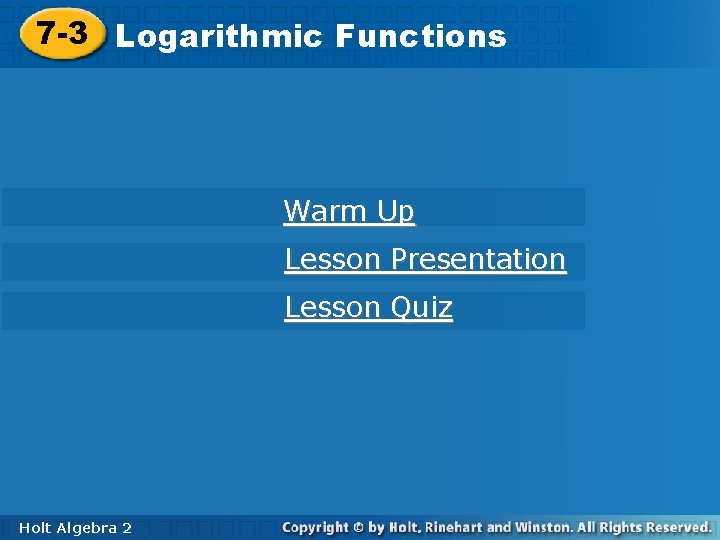7 -3 Logarithmic. Functions Warm Up Lesson Presentation Lesson Quiz Holt Algebra 227 -3 Logarithmic Functions Warm Up Use mental math to evaluate. 1. 4– 3 3. 10– 5 2. 0. 00001 2 4. 5. A power has a base of – 2 and exponent of 4. Write and evaluate the power. (– 2)4 = 16 Holt Algebra 2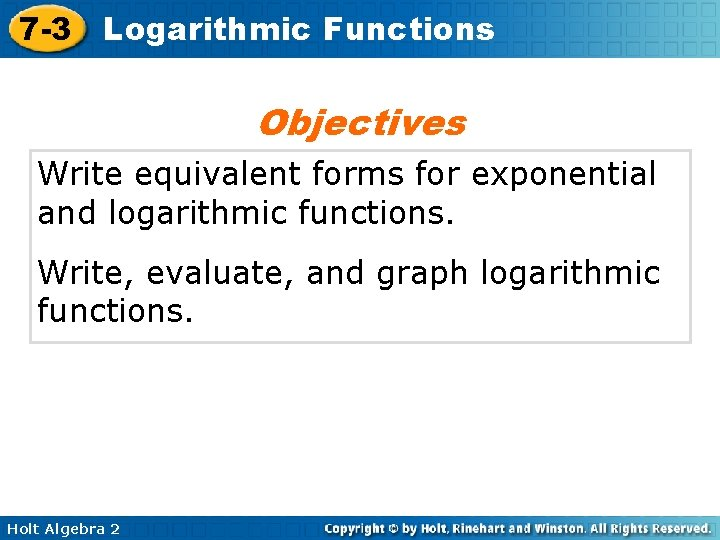7 -3 Logarithmic Functions Objectives Write equivalent forms for exponential and logarithmic functions. Write, evaluate, and graph logarithmic functions. Holt Algebra 2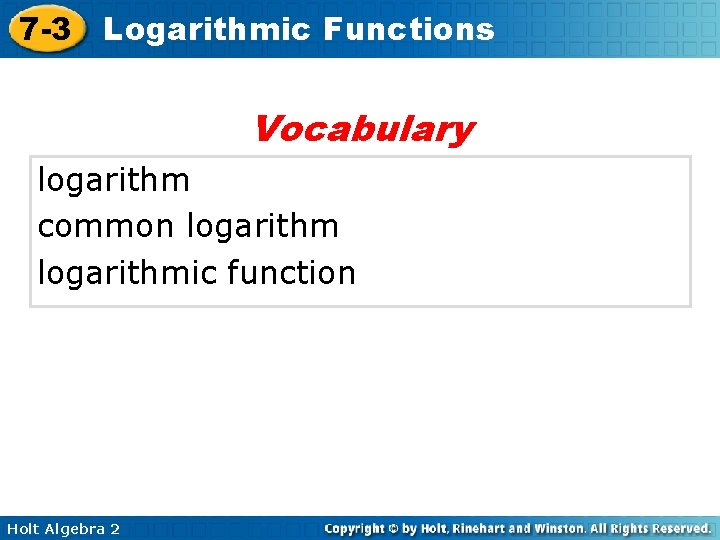7 -3 Logarithmic Functions Vocabulary logarithm common logarithmic function Holt Algebra 27 -3 Logarithmic Functions How many times would you have to double \$1 before you had \$8? You could use an exponential equation to model this situation. 1(2 x) = 8. You may be able to solve this equation by using mental math if you know 23 = 8. So you would have to double the dollar 3 times to have \$8. Holt Algebra 2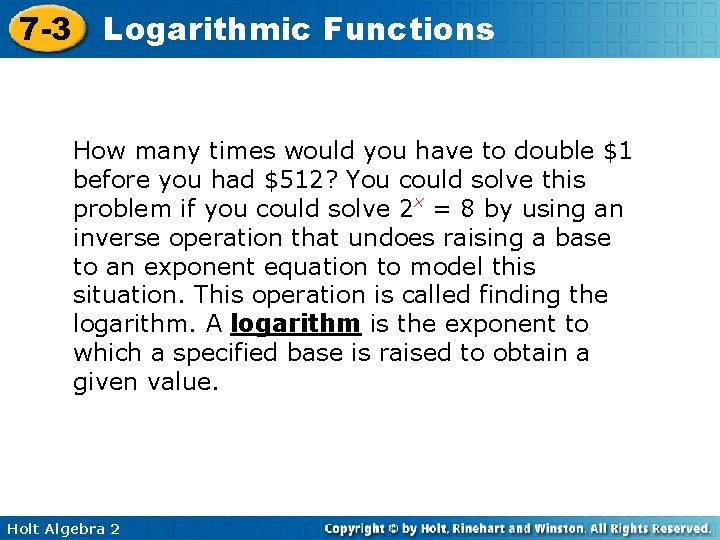7 -3 Logarithmic Functions How many times would you have to double \$1 before you had \$512? You could solve this problem if you could solve 2 x = 8 by using an inverse operation that undoes raising a base to an exponent equation to model this situation. This operation is called finding the logarithm. A logarithm is the exponent to which a specified base is raised to obtain a given value. Holt Algebra 2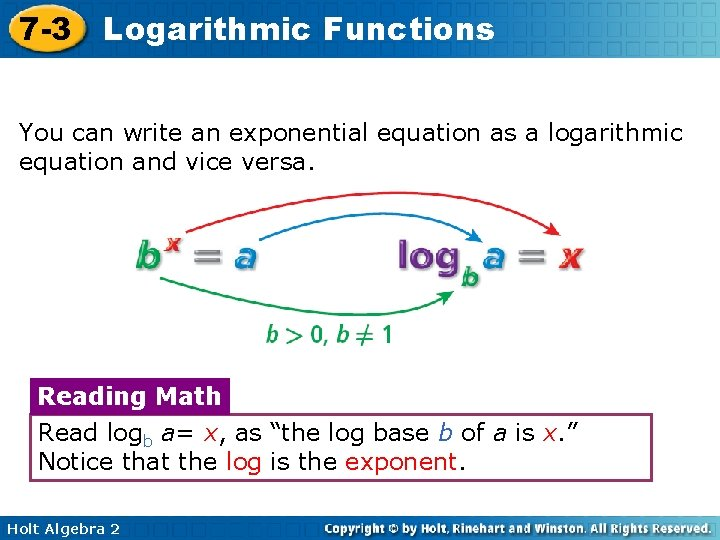7 -3 Logarithmic Functions You can write an exponential equation as a logarithmic equation and vice versa. Reading Math Read logb a= x, as “the log base b of a is x. ” Notice that the log is the exponent. Holt Algebra 27 -3 Logarithmic Functions Example 1: Converting from Exponential to Logarithmic Form Write each exponential equation in logarithmic form. Exponential Equation Logarithmic Form 35 = 243 log 3243 = 5 1 2 25 = 5 log 255 = 104 = 10, 000 log 1010, 000 = 4 6– 1 = ab = c Holt Algebra 2 1 6 log 6 1 6 = – 1 logac =b The base of the exponent becomes the base of the logarithm. The exponent is the logarithm. An exponent (or log) can be negative. The log (and the exponent) can be a variable.7 -3 Logarithmic Functions Check It Out! Example 1 Write each exponential equation in logarithmic form. Exponential Equation Logarithmic Form a. 9 = 81 log 981 = 2 The base of the exponent becomes the base of the logarithm. b. 33 = 27 log 327 = 3 The exponent of the logarithm. logx 1 = 0 The log (and the exponent) can be a variable. 2 0 c. x = 1(x ≠ 0) Holt Algebra 2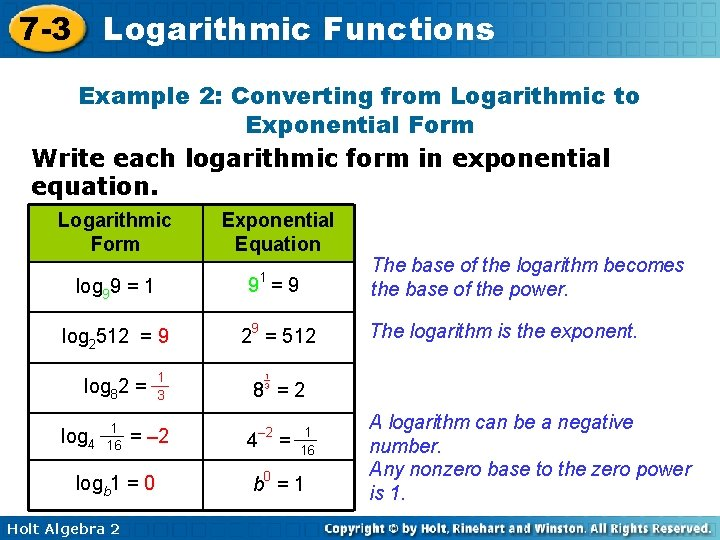7 -3 Logarithmic Functions Example 2: Converting from Logarithmic to Exponential Form Write each logarithmic form in exponential equation. Logarithmic Form Exponential Equation 1 log 99 = 1 9 =9 log 2512 = 9 29 = 512 log 82 = log 4 1 16 = – 2 logb 1 = 0 Holt Algebra 2 1 3 The base of the logarithm becomes the base of the power. The logarithm is the exponent. 1 3 8 =2 4 – 2 = 1 16 b 0 = 1 A logarithm can be a negative number. Any nonzero base to the zero power is 1.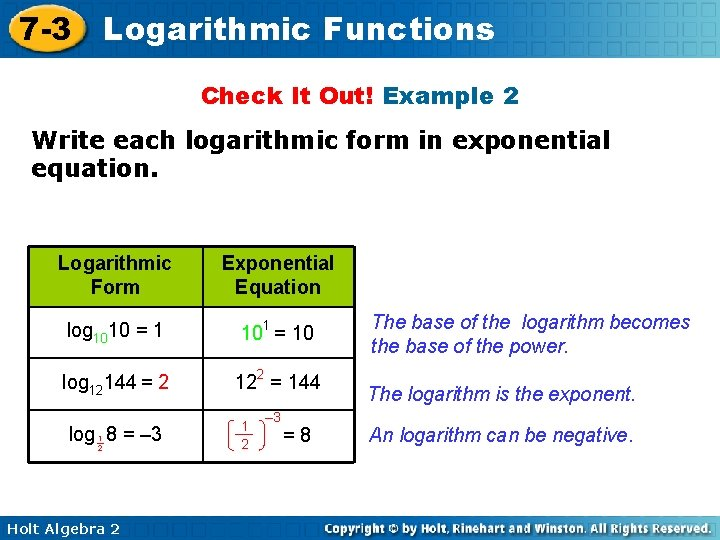7 -3 Logarithmic Functions Check It Out! Example 2 Write each logarithmic form in exponential equation. Logarithmic Form Exponential Equation log 1010 = 1 101 = 10 log 12144 = 2 122 = 144 log 8 = – 3 1 2 Holt Algebra 2 1 2 – 3 =8 The base of the logarithm becomes the base of the power. The logarithm is the exponent. An logarithm can be negative.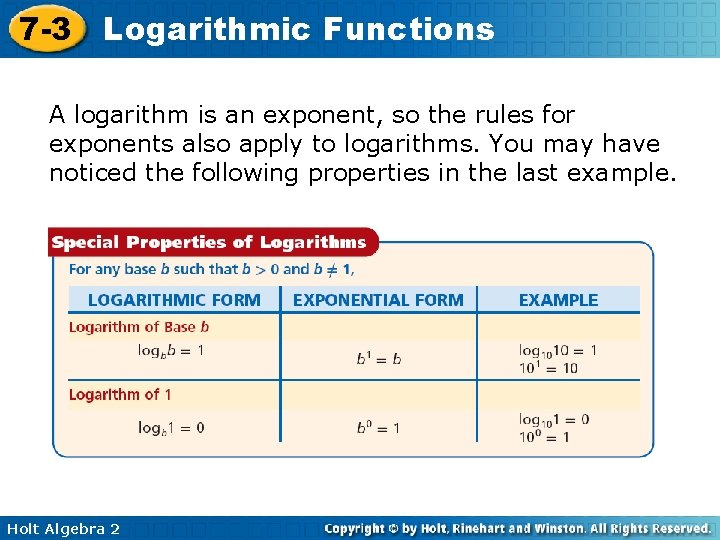7 -3 Logarithmic Functions A logarithm is an exponent, so the rules for exponents also apply to logarithms. You may have noticed the following properties in the last example. Holt Algebra 2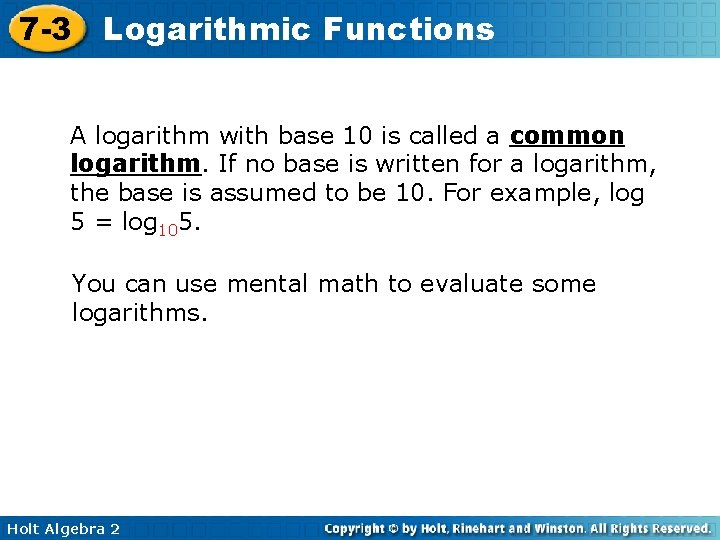7 -3 Logarithmic Functions A logarithm with base 10 is called a common logarithm. If no base is written for a logarithm, the base is assumed to be 10. For example, log 5 = log 105. You can use mental math to evaluate some logarithms. Holt Algebra 2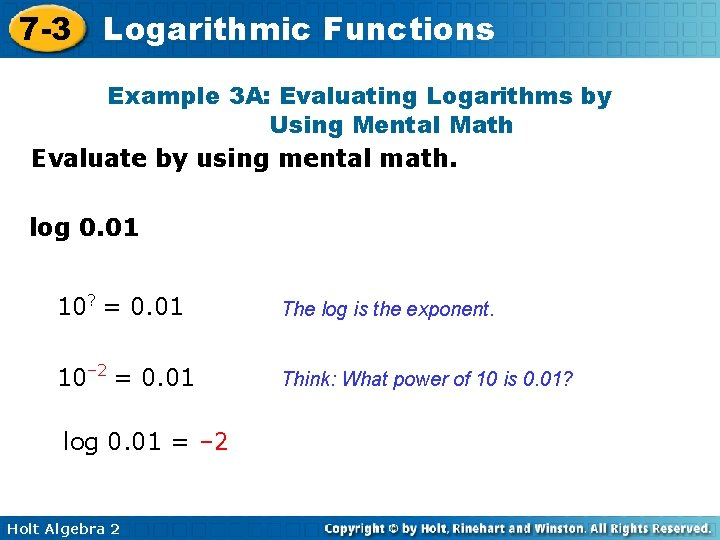7 -3 Logarithmic Functions Example 3 A: Evaluating Logarithms by Using Mental Math Evaluate by using mental math. log 0. 01 10? = 0. 01 The log is the exponent. 10– 2 = 0. 01 Think: What power of 10 is 0. 01? log 0. 01 = – 2 Holt Algebra 2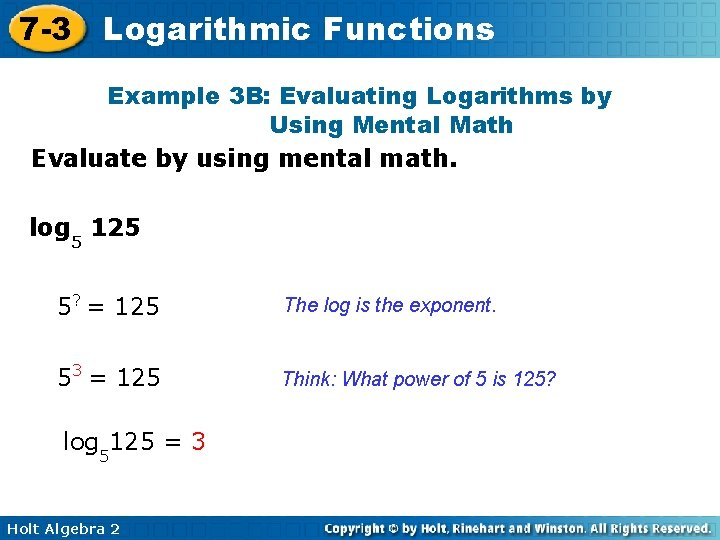7 -3 Logarithmic Functions Example 3 B: Evaluating Logarithms by Using Mental Math Evaluate by using mental math. log 5 125 5? = 125 The log is the exponent. 53 = 125 Think: What power of 5 is 125? log 5125 = 3 Holt Algebra 27 -3 Logarithmic Functions Example 3 C: Evaluating Logarithms by Using Mental Math Evaluate by using mental math. log 5 1 5 5? = 1 5 – 1 5 = 1 log 5 5 Holt Algebra 2 The log is the exponent. 1 5 Think: What power of 5 is 1 ? 5 = – 1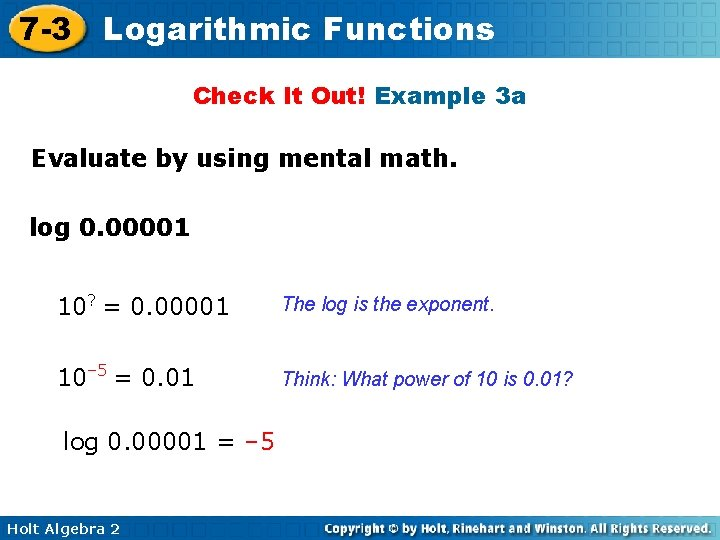7 -3 Logarithmic Functions Check It Out! Example 3 a Evaluate by using mental math. log 0. 00001 10? = 0. 00001 The log is the exponent. 10– 5 = 0. 01 Think: What power of 10 is 0. 01? log 0. 00001 = – 5 Holt Algebra 2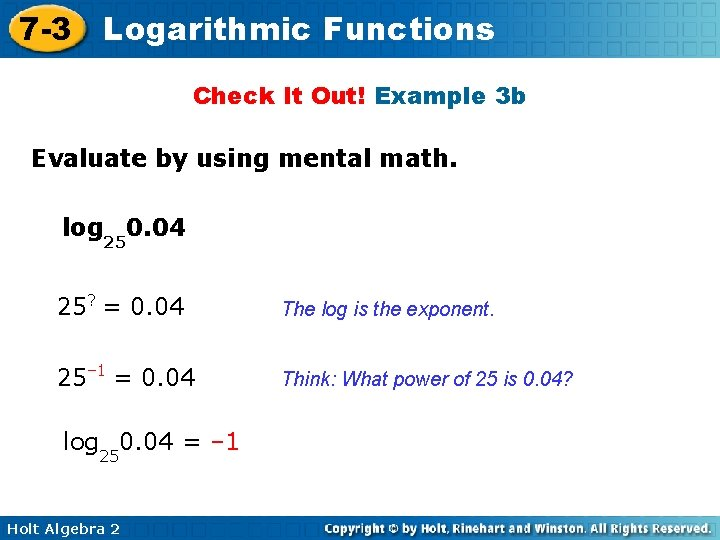7 -3 Logarithmic Functions Check It Out! Example 3 b Evaluate by using mental math. log 250. 04 25? = 0. 04 The log is the exponent. 25– 1 = 0. 04 Think: What power of 25 is 0. 04? log 250. 04 = – 1 Holt Algebra 2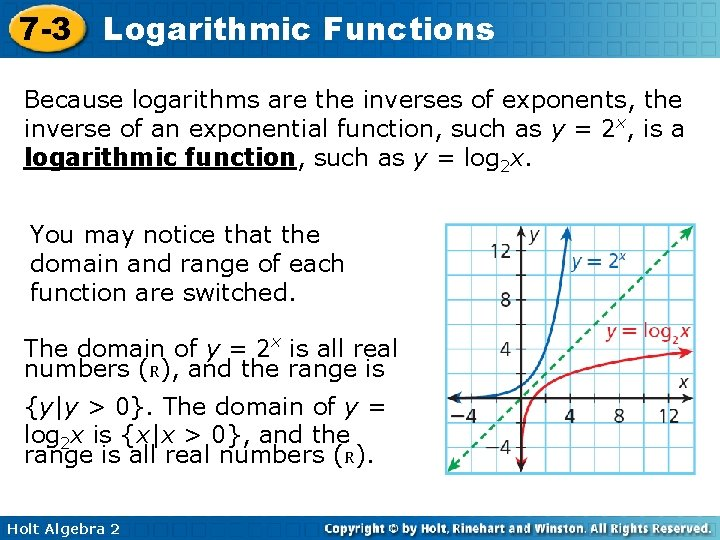7 -3 Logarithmic Functions Because logarithms are the inverses of exponents, the inverse of an exponential function, such as y = 2 x, is a logarithmic function, such as y = log 2 x. You may notice that the domain and range of each function are switched. The domain of y = 2 x is all real numbers (R), and the range is {y|y > 0}. The domain of y = log 2 x is {x|x > 0}, and the range is all real numbers (R). Holt Algebra 2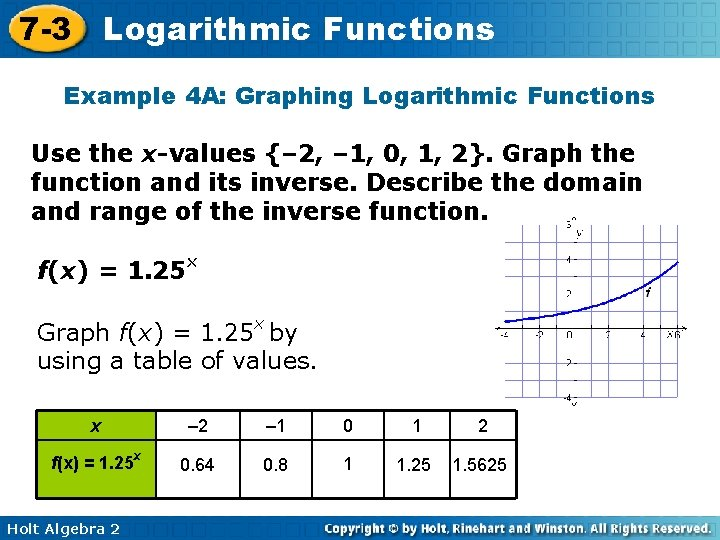7 -3 Logarithmic Functions Example 4 A: Graphing Logarithmic Functions Use the x-values {– 2, – 1, 0, 1, 2}. Graph the function and its inverse. Describe the domain and range of the inverse function. f(x) = 1. 25 x Graph f(x) = 1. 25 x by using a table of values. x – 2 – 1 0 1 2 f(x) = 1. 25 x 0. 64 0. 8 1 1. 25 1. 5625 Holt Algebra 2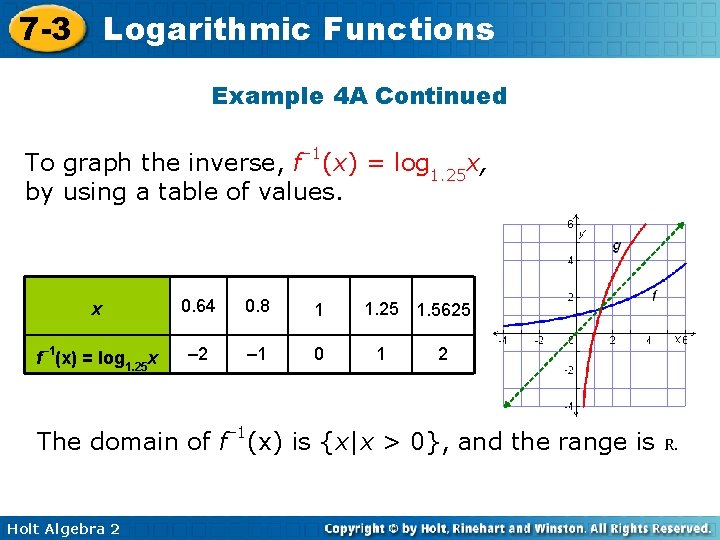7 -3 Logarithmic Functions Example 4 A Continued To graph the inverse, f– 1(x) = log 1. 25 x, by using a table of values. x 0. 64 0. 8 1 f– 1(x) = log 1. 25 x – 2 – 1 0 1. 25 1. 5625 1 2 The domain of f– 1(x) is {x|x > 0}, and the range is R. Holt Algebra 2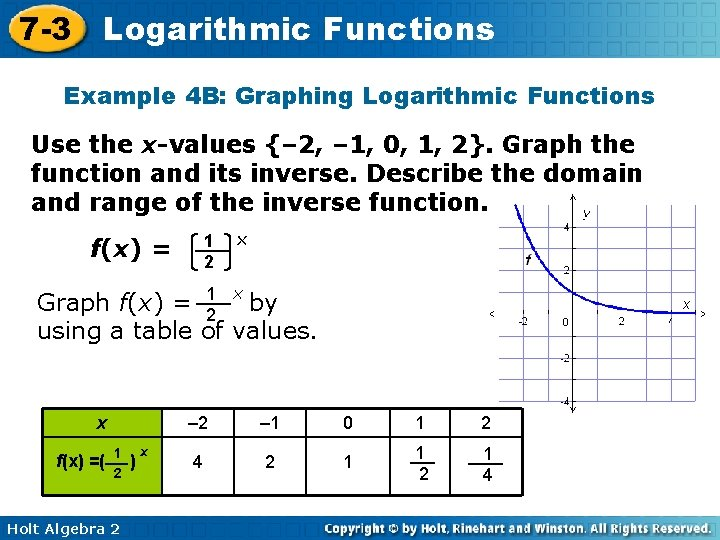7 -3 Logarithmic Functions Example 4 B: Graphing Logarithmic Functions Use the x-values {– 2, – 1, 0, 1, 2}. Graph the function and its inverse. Describe the domain and range of the inverse function. 1 2 f(x) = x Graph f(x) = 12 x by using a table of values. x f(x) =( 1 2 Holt Algebra 2 ) x – 2 – 1 0 1 2 4 2 1 1 2 1 47 -3 Logarithmic Functions Example 4 B Continued To graph the inverse, f– 1(x) = log 1 x, by using a table of 2 values. x f – 1(x) =log 1 2 x 4 2 1 – 2 – 1 0 1 2 1 1 4 2 The domain of f– 1(x) is {x|x > 0}, and the range is R. Holt Algebra 27 -3 Logarithmic Functions Check It Out! Example 4 Use x = – 2, – 1, 1, 2, and 3 to graph . Then graph its inverse. Describe the domain and range of the inverse function. Graph by using a table of values. x f(x) = Holt Algebra 2 3 x 4 – 2 – 1 1 2 3 16 9 4 3 3 4 9 16 27 647 -3 Logarithmic Functions Check It Out! Example 4 To graph the inverse, f– 1(x) = log 3 x, by 4 using a table of values. x 16 9 4 3 3 4 9 16 27 64 f– 1(x) = log 3 x – 2 – 1 1 2 3 4 The domain of f– 1(x) is {x|x > 0}, and the range is R. Holt Algebra 2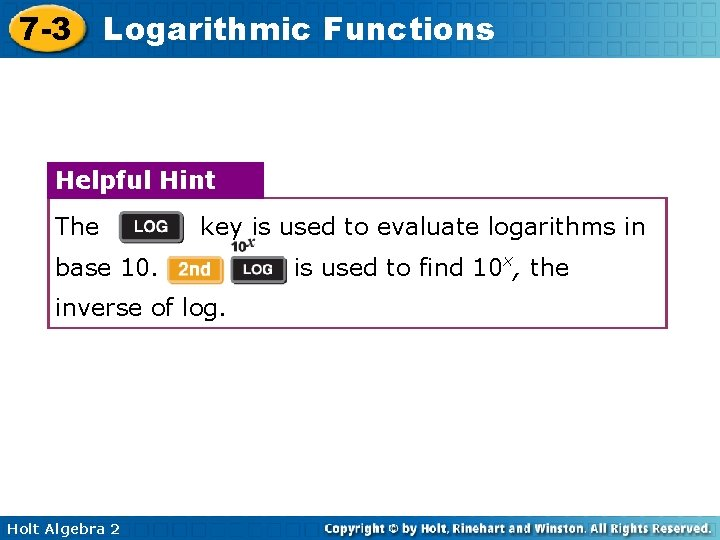7 -3 Logarithmic Functions Helpful Hint The key is used to evaluate logarithms in base 10. inverse of log. Holt Algebra 2 is used to find 10 x, the7 -3 Logarithmic Functions Example 5: Food Application The table lists the hydrogen ion concentrations for a number of food items. Find the p. H of each. Substance Holt Algebra 2 H+ conc. (mol/L) Milk 0. 00000025 Tomatoes 0. 0000316 Lemon juice 0. 0063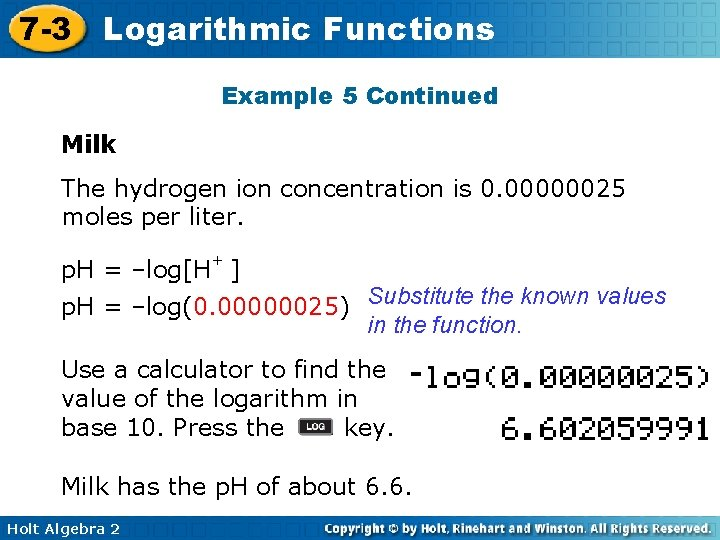7 -3 Logarithmic Functions Example 5 Continued Milk The hydrogen ion concentration is 0. 00000025 moles per liter. p. H = –log[H+ ] p. H = –log(0. 00000025) Substitute the known values in the function. Use a calculator to find the value of the logarithm in base 10. Press the key. Milk has the p. H of about 6. 6. Holt Algebra 2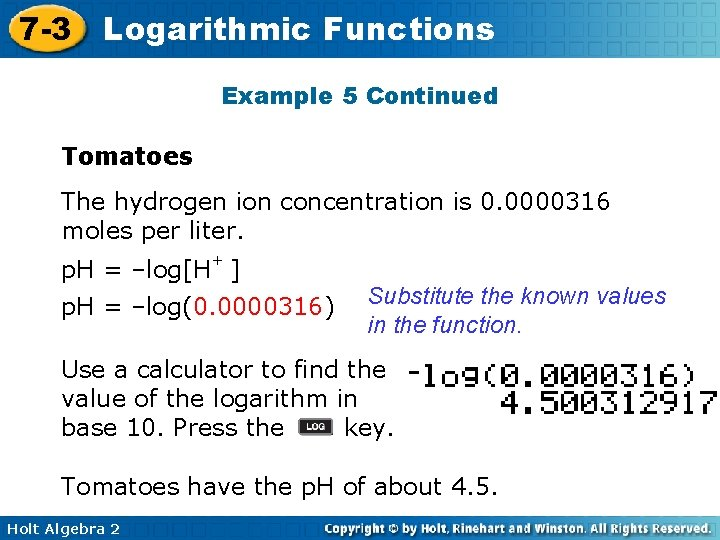7 -3 Logarithmic Functions Example 5 Continued Tomatoes The hydrogen ion concentration is 0. 0000316 moles per liter. p. H = –log[H+ ] p. H = –log(0. 0000316) Substitute the known values in the function. Use a calculator to find the value of the logarithm in base 10. Press the key. Tomatoes have the p. H of about 4. 5. Holt Algebra 27 -3 Logarithmic Functions Example 5 Continued Lemon juice The hydrogen ion concentration is 0. 0063 moles per liter. p. H = –log[H+ ] p. H = –log(0. 0063) Substitute the known values in the function. Use a calculator to find the value of the logarithm in base 10. Press the key. Lemon juice has the p. H of about 2. 2. Holt Algebra 2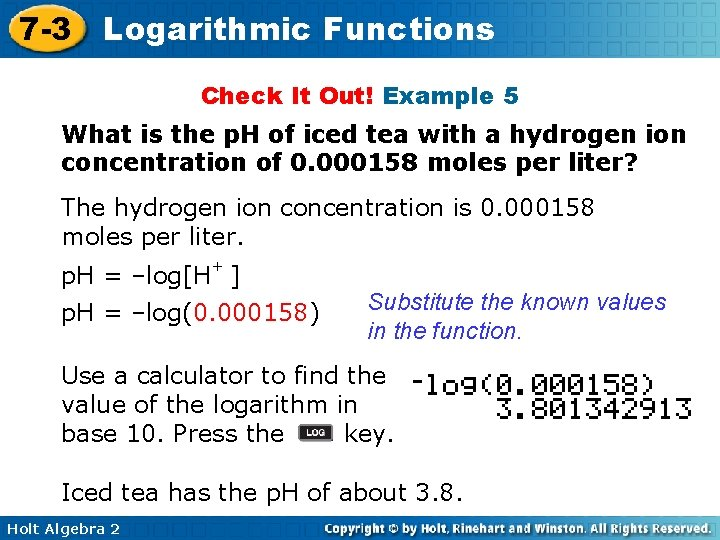7 -3 Logarithmic Functions Check It Out! Example 5 What is the p. H of iced tea with a hydrogen ion concentration of 0. 000158 moles per liter? The hydrogen ion concentration is 0. 000158 moles per liter. p. H = –log[H+ ] p. H = –log(0. 000158) Substitute the known values in the function. Use a calculator to find the value of the logarithm in base 10. Press the key. Iced tea has the p. H of about 3. 8. Holt Algebra 2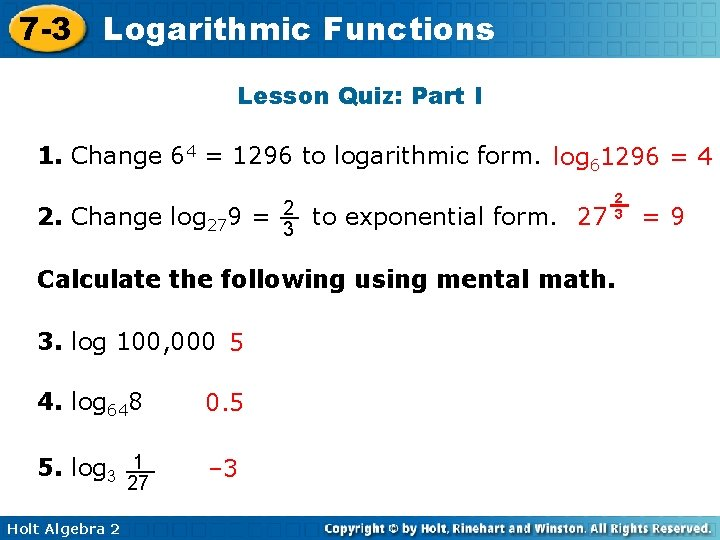7 -3 Logarithmic Functions Lesson Quiz: Part I 1. Change 64 = 1296 to logarithmic form. log 61296 = 4 2. Change log 279 = 2 3 to exponential form. 27 2 3 Calculate the following using mental math. 3. log 100, 000 5 4. log 648 0. 5 5. log 3 1 – 3 27 Holt Algebra 2 =97 -3 Logarithmic Functions Lesson Quiz: Part II 6. Use the x-values {– 2, – 1, 0, 1, 2, 3} to graph f(x) =( 54 )X. Then graph its inverse. Describe the domain and range of the inverse function. D: {x > 0}; R: all real numbers Holt Algebra 2